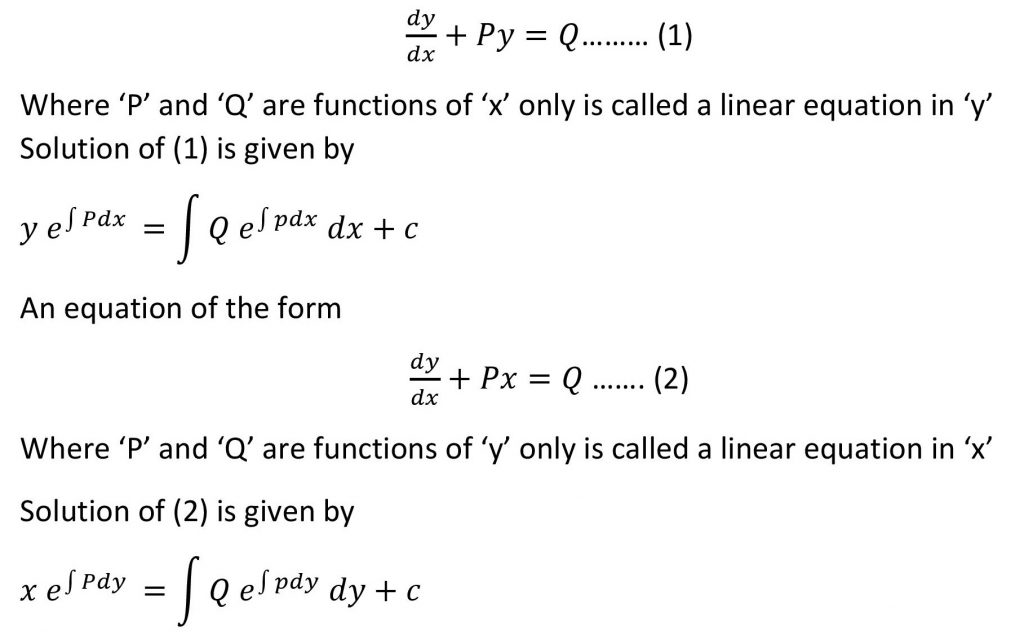### Standard form of a linear equation and its solution

A differential equation is said to be linear if the dependent variable and its derivative occurs in the first degree only and they are not multiplied together.

A differential equation of the form## Bernoulli’s differential equation

The Differential equation of the formWhere ‘P’ and ‘Q’ are functions of ‘x’ is called Bernoulli’s differential equation in ‘y’, then divide the entire equation by  and then by using variable substitution method convert it into a linear equation in ‘t’ and then find the solution .